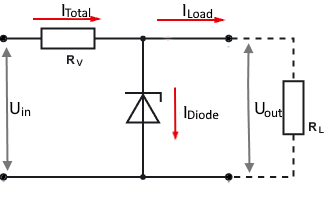# Zener diode voltage regulator calculator

Zener diode voltage regulator calculator for circuits with a fixed load current

### Calculate Series Resistor

This calculator calculates the series resistance of a Zener diode.

The resistance must be dimensioned that the total current does not pull down the output voltage.

A minimal current has to flow through the Zener diode for the stabilization to work. As a guide value, 10% of the load current or 10 mA can be assumed. A value of 10mA is automatically set; it can be overwritten if another value is preferred.

Series Resistor Calculator

 Input Input voltage V Output voltage V .mode { width: 148px; height: 28px; } Load current Load resistor µA mA A Ω kΩ MΩ Diode current µA mA A Decimal places 0 1 2 3 4 6 8 10 Resultat Series resistror Resistor power Diode power Total current## Formulas and description

The total current of the circuit is determined by the series resistor and is always constant. When the load current decreases, the current through the Zener diode increases accordingly.

If the load current fluctuates between maximum and zero, the Zener diode must be dimensioned in such a way that that it can absorb the maximum current and power.

The calculator above assumes a constant load current. If the load current is not constant and falls below it, the Zener diode can overheat and be destroyed.

For circuits with varying loads, use the variable current calculator.

 Total current $$\displaystyle I_{ges}=I_{Z\space min} + I_{L}$$ Diode current $$\displaystyle I_{D}= I_{L} · 0.1$$ Series resistor $$\displaystyle R_v=\frac{U_{ges} - U_Z}{I_{ges}}$$ Power of series resistor $$\displaystyle P_v=(U_{ges} - U_Z) · I_{ges}$$ Power of the diode (variable load current) $$\displaystyle P_D= U_Z · (I_{max} - I_{min} + I_D)$$ Power of the diode (fix load current) $$\displaystyle P_D= U_Z · I_D$$